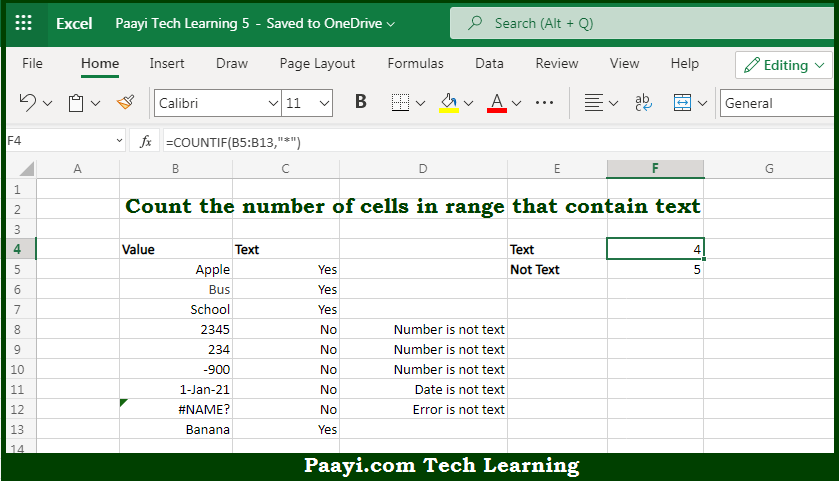# Learn How to Count Cells that Contain Text in Microsoft Excel

Written by | 0 Comments | 339 Views

In this article, you will learn how to COUNT various things in Microsoft Excel using a single or combination of functions and its purpose in Microsoft Excel. You will also get to know how to count cells that contain text and see the generic formula.

Count Cells that Contain Text in Microsoft Excel

The main purpose of this formula is to count the number of cells that contain text. Here we will learn how to count the range of cells containing the text in Microsoft Excel.  That implies, with the help of a formula based on the  COUNTIF function with a wildcard you can able to count the number of cells in a range that contains text that means - not numbers, not errors, not blank. The generic form of the formula provided below "rng" represents a range of cells. So, with the help of this formula, you can able to count the number of cells that contain the text.

General Formula to COUNT Cells that Contain Text

=COUNTIF(range,"*")

The Explanation for the COUNT Cells that Contain TextSo we know that with the help of the given formula above you can able to count the number of cells that contain text. Here we will learn how to count the range of cells containing the text in Microsoft Excel. As we know that, the  COUNTIF function is used to count cells in a range that contains the text. In the example provided here, the solution uses the COUNTIF function, which counts the number of cells that match the provided criteria. Here, the criteria are supplied as an asterisk (*), which is the wildcard that matches any number of text characters. So, with the help of this formula, you can able to count cells that contain text.